# Microsoft Excel – Data Validation Functions and Conditional Formatting

Microsoft Excel has numerous functions and features that are used to validate data and apply conditional formatting to cells. Below we’ll see how to combine these features along with the CODE, ISNUMBER, and LEN functions to make quick work of reviewing and validating user data before, in my case, moving that content into SharePoint lists. It’s a simple and cost effective way of performing content reviews without taking on the overhead of fixing up content after an import.

Recently I was working with user codes that were in the format of: capitalized letter of last-name followed by the zip code. For example, Kat Sanchez from Chicago would be S60628. There were about 600 entries that looked something like this sample:Some of the anomalies obviously stick out (e.g. A7), but among 500+ entries even cells like A7 are hard to spot, and I don’t want to spend time filtering/sorting. The fact is: I can write a simple formula to perform my data validation on every cell and then apply conditional formatting to REALLY make the erroneous content stand-out. Let’s look at how this is done.

# Microsoft Excel CODE Function

The CODE() function evaluates the first character of a specified cell and compares it to an ASCII character code. For my exercise, the first character of any cell must be between A-Z – that’s capital A through Z. Those characters fit exactly in the ASCII code range of 65 – 90. So, to start out, let’s look at the results of the formula

`=AND(CODE(\$A1) >= 65,CODE(\$A1) <=90)`

Here are the results against a small sample set:All cells show TRUE except A7, A8, A9, and A11. This is functioning as expected.

# Microsoft Excel LEN Function

All entries are expected to be 6 characters in length. That is, for example, B60628 or W60647. Here we use a formula:

`=LEN(\$A1)=6`

Results are as expected – formula looks good:# Microsoft Excel ISNUMBER Function

The final component of our validation formula is that the second-through-sixth characters must be numeric. That is:

`=ISNUMBER(VALUE(MID(\$A1,2,5)))`

I know, there’s actually more than the ISNUMBER function here. I don’t want to get into too many details on the VALUE function or the MID function, but briefly:

• The VALUE function will return a text value converted to a number or #VALUE! if specified content is not a number.
• The MID function returns characters from the starting point of the first argument, extending out for ‘n’ characters from the second argument.

So, here too we see what’s expected: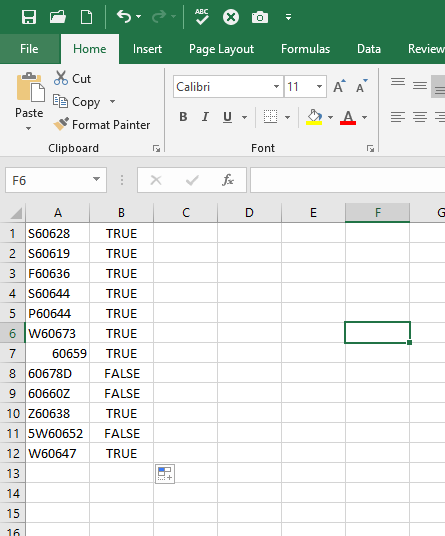However, the real key is combining all of this together using the AND function.

# Final Formula using the AND Function

Logically written, our function reads something to the tune of: The value must start with a capital letter, followed by 5 numbers, which equals exactly 6 characters.

So, that’s:

`=AND(CODE(\$A1)>=65,CODE(\$A1)<=90,ISNUMBER(VALUE(MID(\$A1,2,5))),LEN(\$A1)=6)`

There we go:# Conditional Formatting

One way to tackle identifying  “TRUE” or “FALSE” values would be to add filtering. But, in this case, I need to see the entire data set to help with copy-and-paste, sorting, and other business requirements. So, filtering to only “TRUE” or “FALSE” values is not acceptable. Instead what I can do is use Microsoft Excel Conditional Formatting features to very easily make “TRUE” values obvious.

The first thing I’ll do is highlight the column, and click Home > Conditional Formatting, Highlight Cell Rules > Equal To…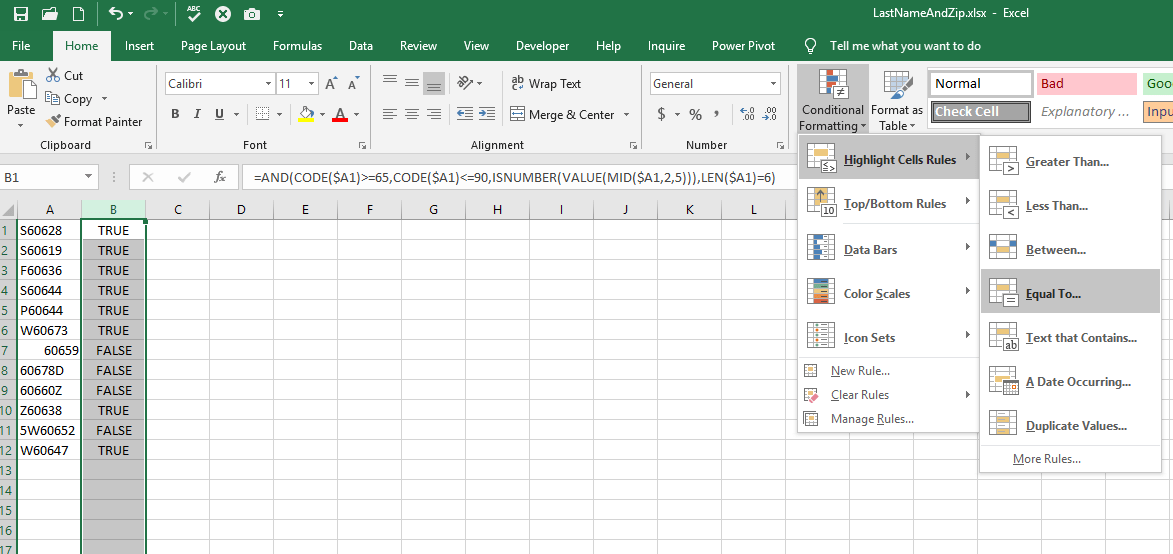Then, I simply specify the following: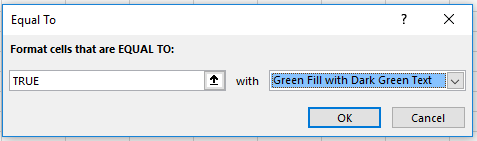And this results in: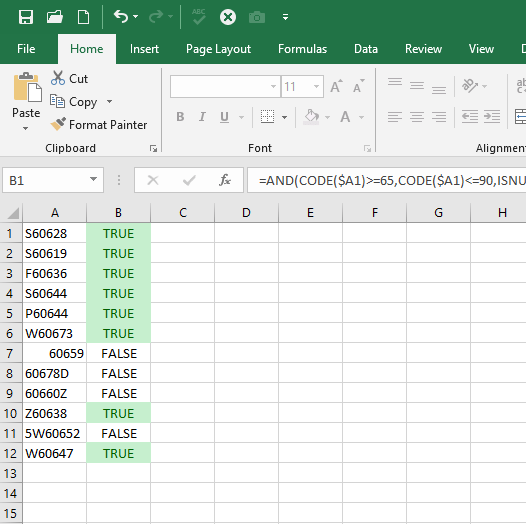It’s now very obvious which values I need to focus on and work with the data provider on fixing.

# Final Note On Data Validation

Briefly, I want to call-out that there are also features and functions focused specifically on Data Validation: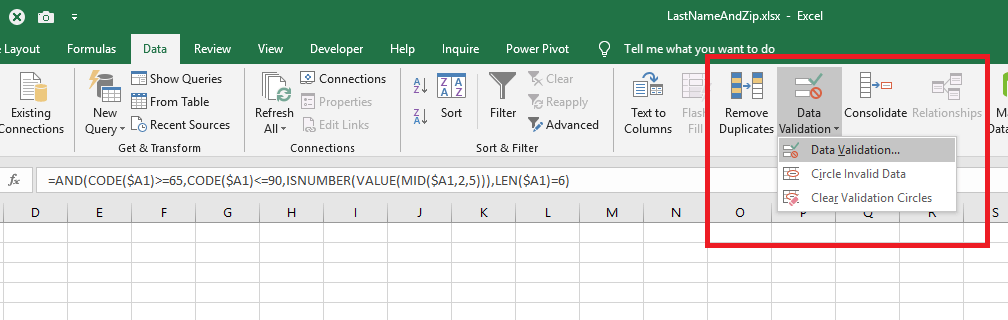You can take the formula you see in the formula bar, move it into the Data Validation specifications, and expose a whole new set of options for finding, evaluating, and resolving data validation issues. In this post though, my intent was only to show a quick and easy way for displaying data validation errors using easy-to-identify visualizations before moving the content elsewhere (e.g. SharePoint or SQL). The full-fledged data validation functions are better suited for scenarios where the workbook itself is where you intend to manage the content…so, I’ll cover that topic in a future post.